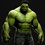# Interesting Integral

I am currently preparing for IITJEE 2017.After class, my teacher gave me a sheet with a few indefinite integral questions.We got most of the questions but we couldn't solve one question.Nobody could solve it, which is when I decided to discuss about this problem online.The question is,

Integrate: $\int$$\frac{ln(1+x^{2})}{\sqrt{1-x^{2}}}$$dx$

I substituted $x$ = $\sin \theta$ but I finally got an expression like

$\int$$\ln(1+\sin^{2} \theta)$$d \theta$

Then I converted $\sin^{2} \theta$ to $1-\cos^{2} \theta$ and applied the formula $a^{2}-b^{2}$ = $(a-b)(a+b)$

At last I got , $\int$$ln(\sqrt{2}-\cos \theta)$$d \theta$ $+$ $\int$$ln(\sqrt{2}+\cos \theta)$$d \theta$

After this I got stuck.I tried many methods but I couldn't get a perfect solution.

I usually go to classes in morning.So if anyone replies during that time I won't be able to respond.I come home by 8pm. After that I can reply.Please post the solution if anyone has got a different method than mine.Note by Dhanvanth Balakrishnan
4 years, 5 months ago

This discussion board is a place to discuss our Daily Challenges and the math and science related to those challenges. Explanations are more than just a solution — they should explain the steps and thinking strategies that you used to obtain the solution. Comments should further the discussion of math and science.

When posting on Brilliant:

• Use the emojis to react to an explanation, whether you're congratulating a job well done , or just really confused .
• Ask specific questions about the challenge or the steps in somebody's explanation. Well-posed questions can add a lot to the discussion, but posting "I don't understand!" doesn't help anyone.
• Try to contribute something new to the discussion, whether it is an extension, generalization or other idea related to the challenge.
• Stay on topic — we're all here to learn more about math and science, not to hear about your favorite get-rich-quick scheme or current world events.

MarkdownAppears as
*italics* or _italics_ italics
**bold** or __bold__ bold
- bulleted- list
• bulleted
• list
1. numbered2. list
1. numbered
2. list
Note: you must add a full line of space before and after lists for them to show up correctly
paragraph 1paragraph 2

paragraph 1

paragraph 2

[example link](https://brilliant.org)example link
> This is a quote
This is a quote
    # I indented these lines
# 4 spaces, and now they show
# up as a code block.

print "hello world"
# I indented these lines
# 4 spaces, and now they show
# up as a code block.

print "hello world"
MathAppears as
Remember to wrap math in $$ ... $$ or $ ... $ to ensure proper formatting.
2 \times 3 $2 \times 3$
2^{34} $2^{34}$
a_{i-1} $a_{i-1}$
\frac{2}{3} $\frac{2}{3}$
\sqrt{2} $\sqrt{2}$
\sum_{i=1}^3 $\sum_{i=1}^3$
\sin \theta $\sin \theta$
\boxed{123} $\boxed{123}$

## Comments

There are no comments in this discussion.

×

Problem Loading...

Note Loading...

Set Loading...